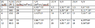# Graphing the Results from Kinetics Experiments with Enzyme Inhibitors

Sara1bara

## Homework Statement

The following kinetic data were obtained for an enzyme in the absence of any inhibitor (1), and in the presence of two different inhibitors (2) and (3) at 5mM concentration. Assume [ET] is the same in each experiment[/B]
(1) (2) (3)
v (umol/mL * sec) v (umol/mL * sec) v (umol/mL * sec)
(mM)
1 12 4.3 5.5
2 20 8 9

4 29 14 13
8 35 21 16
12 40 26 18

Graph these data as Lineweaver-Burk plots and use your graph to find answers to a and b

a. Determine Vmax & Km for the enzyme
b. Determine the type of inhibition and the KI for each inhibitor

## Homework Equations

1/Vmax = y-intercept, slope = km/Vmax

## The Attempt at a Solution

See attached table*[/B]
This is a table of above plus the reciprocal of each one. It is from the answer key of the homework, however for 1/ instead of the answer being 1, they change the units to M^-1. Similarly for V the units are mL*sec/umol and 1/V the units are mL*sec/umol. I do not know why they change the units and it confuses me on how to get all of the numbers.

From there, I made a graph, one for each of the inhibitors. X was the 1/ value and Y was the 1/V value.

From there, I was unsure how to find the slope and y-intercept since the numbers are not clear cut. I used the formula 1/V = Km/Vmax X 1/ + 1/Vmax to understand the relationship between y-intercept and slope. The answer key explained the formulas for each of the inhibitors making it possible for me to understand how to calculate Vmax and Km by knowing that 1/Vmax was the y-intercept and that the slope was Km/Vmax.

The answer key showed these equations:

1. 1/V = 63.1 (1/[S}) + 1.96 X 10^4
2. 1/V = 212.3 (1/) + 1.99 X 10 ^4
3. 1/V = 137.4 (1/) + 4.38 X 10^4

From here I calculated the Km and Vmax, but again was confused with units.
I also tried calculating the slope via the equation y2-y1/x2-x1 using two points, but was off compared to the answer key.

For part b. I worked with my knowledge of the different types of inhibitors to know which formula to use

Competitive : Km,app = Km(1 + I/KI)
Noncompetitive: 1/Vmax,app = 1/Vmax (1 + I/KI)

It was hard for me to know which numbers to plug in here as part a and the introduction to the problem were also confusing.

#### Attachments

•Screen Shot 2014-11-15 at 7.01.11 PM.png
9.7 KB · Views: 671